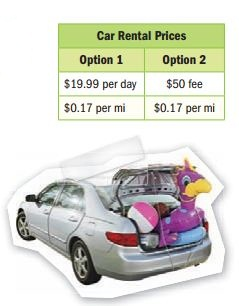Homework Explained - Math Practice 101Dear guest, you are not a registered member. As a guest, you only have read-only access to our books, tests and other practice materials.

As a registered member you can:

Registration is free and doesn't require any type of payment information. Click here to Register.
Go to page:
Chapter 5: Expressions; Lesson 1: Algebraic Expressions

Evaluate each expression if d = 8, e = 3, f = 4, and g = -1.

•  $$2(d+9)=$$
•  $$\large\frac{d}{4}=$$
•  $$\large\frac{ef}{4}=$$
•  $$4f+d=$$
•  $$\large\frac{5d-25}{5}=$$
•  $$d^2+7=$$
•  $$\large\frac{d-4}{2}=$$
•  $$10(e+7)=$$
•  $$\large\frac{2g}{2}=$$
• Question 10

The expression 5n + 2 can be used to find the total cost in dollars of bowling where n is the number of games bowled and 2 represents the cost of shoe rental. How much will it cost Vincent to bowl 3 games?

• Question 11

A car rental company’s fees are shown. Suppose you rent a car using Option 2. Write an expression that gives the total cost in dollars for driving m miles. Then find the cost for driving 150 miles.•  $$\$$
• Question 12

Refer to Exercise 11. Suppose you rent a car using Option 1. Write an expression that gives the total cost in dollars to rent a car for d days and m miles. Then find the cost for renting a car for 2 days and driving 70 miles.

•  \$

Yes, email page to my online tutor. (if you didn't add a tutor yet, you can add one here)